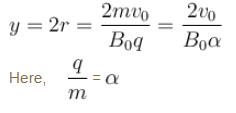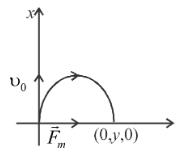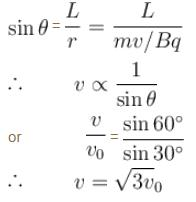Courses

# Magnetic Effect Of Current NAT Level - 2

## 10 Questions MCQ Test Basic Physics for IIT JAM | Magnetic Effect Of Current NAT Level - 2

Description
This mock test of Magnetic Effect Of Current NAT Level - 2 for Physics helps you for every Physics entrance exam. This contains 10 Multiple Choice Questions for Physics Magnetic Effect Of Current NAT Level - 2 (mcq) to study with solutions a complete question bank. The solved questions answers in this Magnetic Effect Of Current NAT Level - 2 quiz give you a good mix of easy questions and tough questions. Physics students definitely take this Magnetic Effect Of Current NAT Level - 2 exercise for a better result in the exam. You can find other Magnetic Effect Of Current NAT Level - 2 extra questions, long questions & short questions for Physics on EduRev as well by searching above.
*Answer can only contain numeric values
QUESTION: 1

### A charged particle enters a uniform magnetic field with velocity vector at an angle of 45° with the magnetic field. The pitch of the helical path followed by the particle is p. The radius of the helix is given by p/nπ. The value of n is

Solution: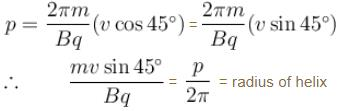*Answer can only contain numeric values
QUESTION: 2

### A copper wire of diameter 1.6 mm  carries a current  i. The maximum magnetic field due to this wire is 5 × 10–3 T.  The value of i  is :

Solution:

Maximum magnetic field is at surface and is given by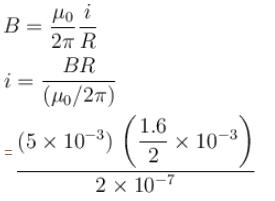20A

*Answer can only contain numeric values
QUESTION: 3

### The magnetic field at the centre of an equilateral triangular loop of side 2L and carrying a current i  is given by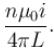Find the value of  n  ____.

Solution:

Magnetic field at O  is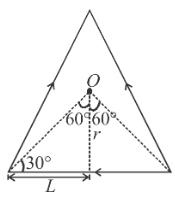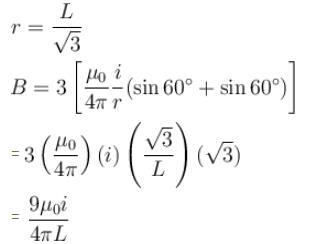*Answer can only contain numeric values
QUESTION: 4

Ratio of magnetic field at the centre of a current carrying coil of radius R and at a distance of 3R on its axis is :

Solution:

The desired ratio is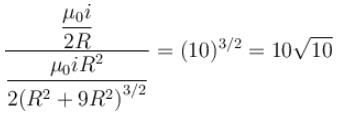*Answer can only contain numeric values
QUESTION: 5

Same current i = 2A  is flowing in a wire frame as shown in figure. The frame is a combination of two equilateral triangles ACD and CDE of side 1m. It is placed in uniform magnetic field  B = 4T acting perpendicular to the plane of frame. The magnitude of magnetic force acting on the frame is :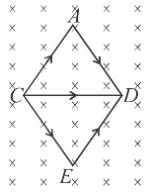Solution: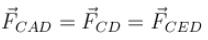∴  Net force on frame =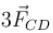= (3) (2) (1) (4)    (F = ilB)
= 24 N

*Answer can only contain numeric values
QUESTION: 6

Equal currents i = 1 A are flowing through the wires parallel to y-axis located at  x = +1mx = +2, x = +4m  etc, but in opposite directions as shown. The magnetic field at origin (in units of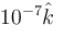tesla) will be :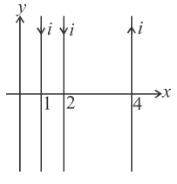Solution: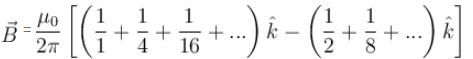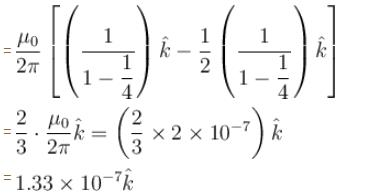*Answer can only contain numeric values
QUESTION: 7

A conducting ring of mass kg  and radius 0.5 m  is placed on a smooth horizontal plane. The ring carries a current i = 4A. A horizontal magnetic field B = 10 T is switched on at time t = 0 as shown in figure. The initial angular acceleration (in rad/s2) of the ring will be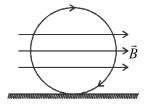Solution: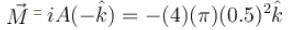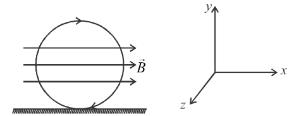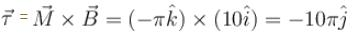Axis of rotation in along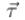i.e., axis of rotation in the y-axis. Moment of inertia about which is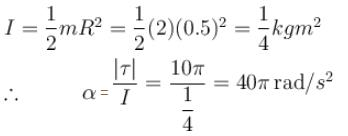*Answer can only contain numeric values
QUESTION: 8

Two parallel wires carrying equal currents in opposite directions are placed at x = +a  parallel to y-axis with z = 0. Magnetic field at origin O is B1 and at P (2a, 0, 0) is  B2. Then the ratio B1/B2  is :

Solution:

Case I : If the currents are in the directions shown in figure. Then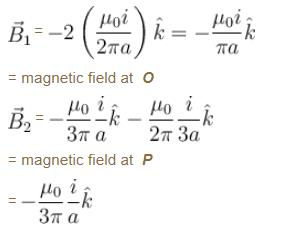∴ B1/B2 = –3
Case II
: In the case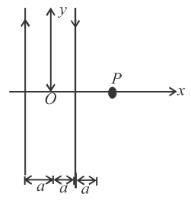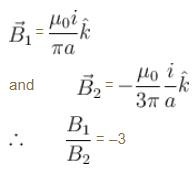*Answer can only contain numeric values
QUESTION: 9

A particle of charge per unit mass α is released from origin with a velocity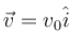in a uniform magnetic field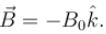If the particle passes through (0, y, 0), then y  is equal to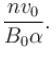The value of n  is ____.

Solution: